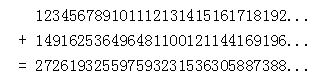Online JudgeProblem SetAuthorsOnline ContestsUser
Web Board
F.A.Qs
Statistical Charts
Problems
Submit Problem
Online Status
Prob.ID:
Register
Authors ranklist
Current Contest
Past Contests
Scheduled Contests
Award Contest
Register
Language:
 Time Limit: 1000MS Memory Limit: 65536K Total Submissions: 1996 Accepted: 422

Description

Let us write down the infinite consecutive integers in a sequence in one line without any space and their squares in the second line. This will generate two different long numbers, now we want to find the sum of these two numbers. The calculation of the first 30 digits is just as below.The first digit of the result is 2, the second digit is 7, and the third is 2 and so on. Given an integer k, you should output the digit at position k in the resulting number.

Input

The input file will contain several test cases. In each of the test cases, there is an integer k (0 < k <= 2 ^ 31 - 1) in one line.

A line containing a number "0" terminates input, and this line need not be processed.

Output

For each test case you should generate a line of output, which is the digit in the k-th place of the addition result.

Sample Input

```2
5
30
0
```

Sample Output

```7
1
8
```

Source

POJ Monthly--2005.07.31, Islamic Azad University of Mashhad – Collegiate Coding Challenge 1

[Submit]   [Go Back]   [Status]   [Discuss]Home PageGo BackTo top